## How to Calculate and Solve for MBAL Gas Solubility | The Calculator Encyclopedia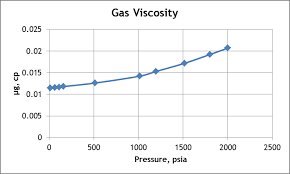The image above represents the MBAL gas solubility.

To compute for the MBAL gas solubility, four essential parameters are needed and these parameters are Specific Gravity of Solution Gas (γg), Specific Gravity of Stock Tank Oil (γo), Oil FVF (Bo) and Oil Density (ρo).

The formula for calculating MBAL gas solubility:

Rs = Boρo – 62.4γo / 0.0136γg

Where:

Rs = MBAL Gas Solubility
γg = Specific Gravity of Solution Gas
γo = Specific Gravity of Stock Tank Oil
Bo = Oil FVF
ρo = Oil Density

Let’s solve an example;
Given that specific gravity of solution gas is 9, specific gravity of stock tank oil is 13, oil FVF is 19 and oil density is 27. Find the MBAL gas solubility?

This implies that;

γg = Specific Gravity of Solution Gas = 9
γo = Specific Gravity of Stock Tank Oil = 13
Bo = Oil FVF = 19
ρo = Oil Density = 27

Rs = Boρo – 62.4γo / 0.0136γg
Rs = 19 x 27 – 62.4 x 13 / 0.0136 x 9
Rs = 513 – 811.19 / 0.1224
Rs = -298.19 / 0.1224
Rs = -2436.27

Therefore, the MBAL gas solubility is -2436.27.

## How to Calculate and Solve for Marhoun’s Gas Solubility in a Fluid | The Calculator Encyclopedia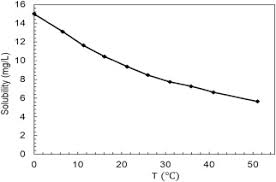The image above represents marhoun’s gas solubility.

To compute for the marhoun’s gas solubility, four essential parameters are needed and these parameters are Gas Specific Gravity (γg), Temperature (°Rankine) (T), Pressure (P) and Stock Tank Oil Gravity (γo).

The formula for calculating the marhoun’s gas solubility:

Rs = [a γgb γoc Td P]e

Where:

Rs = Marhoun’s Gas Solubility
a = 185.843208
b = 1.877840
c = -3.1437
d = -1.32657
e = 1.398441
γg = Gas Specific Gravity
T = Temperature (°Rankine)
P = Pressure
γo = Stock Tank Oil Gravity

Let’s solve an example;
Find the marhoun’s gas solubility when the gas specific gravity is 14, temperature is 80, pressure is 40 and the stock tank oil gravity is 24.

This implies that;

γg = Gas Specific Gravity = 14
T = Temperature (°Rankine) = 80
P = Pressure = 40
γo = Stock Tank Oil Gravity = 24

Rs = [a γgb γoc Td P]e
Rs = [185.843208 x 141.87784 x 24-3.1437 x 80-1.32657 x 40]1.398441
Rs = [185.843208 x 141.98 x 24-3.1437 x 80-1.32657 x 40]1.398441
Rs = [185.843208 x 141.98 x 0.0000458 x 80-1.32657 x 40]1.398441
Rs = [185.843208 x 141.98 x 0.0000458 x 0.00298 x 40]1.398441
Rs = [0.1445]1.398441
Rs = 0.0668

Therefore, the marhoun’s gas solubility is 0.0668.

## How to Calculate and Solve for Glass Gas Solubility Parameter in a Fluid | Nickzom Calculator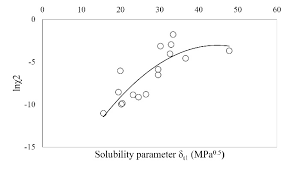The image above represents glass gas solubility parameter.

To compute for the glass gas solubility parameter, one essential parameter is needed and the parameter is Pressure (P).

The formula for calculating the glass gas solubility parameter:

x = 2.8869 – [14.1811 – 3.3093logP]0.5

Where:

x = Glass Gas Solubility Parameter, x
P = Pressure

Let’s solve an example;
Find the glass gas solubility parameter when the pressure is 62.

This implies that;

x = Glass Gas Solubility Parameter, x = 62

x = 2.8869 – [14.1811 – 3.3093logP]0.5
x = 2.8869 – [14.1811 – 3.3093log(62)]0.5
x = 2.8869 – [14.1811 – 3.3093(1.79)]0.5
x = 2.8869 – [14.1811 – 5.93]0.5
x = 2.8869 – [8.249]0.5
x = 2.8869 – 2.87
x = 0.0146

Therefore, the Glass Gas Solubility Parameter is 0.0146.

## How to Calculate and Solve for Glass Gas Solubility in a Fluid | The Calculator Encyclopedia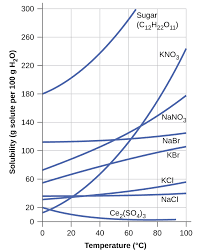The image above represents glass gas solubility.

To compute for the glass gas solubility, four essential parameters are needed and these parameters are API Gravity (°API), Temperature (°Rankine) (T), Mean Bubble Point (Pb*) and Gas Gravity at Actual Separator Psep and Tsepg).

The formula for calculating the glass gas solubility:

γgs = γg[((°API)0.989 / (T – 460)0.172)Pb*]1.2255

Where:

γgs = Glass Gas Solubility
°API = API Gravity
T = Temperature (°Rankine)
Pb* = Mean Bubble Point
γg = Gas Gravity at Actual Separator Psep and Tsep

Let’s solve an example;
Find the glass gas solubility when the API Gravity is 15, the temperature is 30, the mean bubble point is 40 and the gas gravity at actual separator is 54.

This implies that;

°API = API Gravity = 15
T = Temperature (°Rankine) = 30
Pb* = Mean Bubble Point = 40
γg = Gas Gravity at Actual Separator Psep and Tsep = 54

γgs = γg[((°API)0.989 / (T – 460)0.172)Pb*]1.2255
γgs = 54[((15)0.989 / (30 – 460)0.172)40]1.2255
γgs = 54[((15)0.989 / (-430)0.172)40]1.2255
γgs = 54[((15)0.989 / (NaN))40]1.2255
γgs = 54[(14.5 / NaN)40]1.2255
γgs = 54[(NaN)40]1.2255
γgs = 54[NaN]1.2255
γgs = 54[NaN]
γgs = NaN

Therefore, the glass gas solubility is NaN.

## How to Calculate and Solve for Vasquez-Beggs Gas Solubility in a Fluid | The Calculator Encyclopedia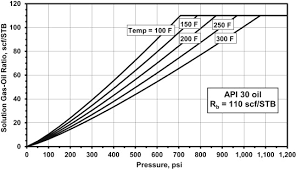The image above represents the Vasquez-Beggs Gas Solubility.

To compute for the Vasquez-Beggs gas solubility, five essential parameters are needed and these parameters are API Gravity (°API), Temperature (°Rankine) (T), Gas Gravity at Reference Separator Pressure (γgs), Gas Gravity at Actual Separator Psep and Tsep g) and Gas Solubility Parameter (C1, C2, C3).

The formula for calculating Vasquez-Beggs gas solubility:

Rs = C1 γgs γgC2 exp[C3(°API / T)]

Where:

Rs = Vasquez-Beggs Gas Solubitity
°API = API Gravity
T = Temperature (°Rankine)
γgs = Gas Gravity at Reference Separator Pressure
γg = Gas Gravity at Actual Separator Psep and Tsep
C1, C2, C3 = Gas Solubility Parameter

Let’s solve an example;
Find the Vasquez-Beggs gas solubility when the API Gravity is 24, Temperature is 180, gas gravity at reference separator pressure is 60, gas gravity at actual separator is 56, gas solubility parameter is 72, 66 and 45.

This implies that;

°API = API Gravity = 24
T = Temperature (°Rankine) = 180
γgs = Gas Gravity at Reference Separator Pressure = 60
γg = Gas Gravity at Actual Separator Psep and Tsep = 56
C1, C2, C3 = Gas Solubility Parameter = 72, 66, 45

Rs = C1 γgs γgC2 exp[C3(°API/ T)]
Rs = 72 x 60 x 5666 exp[45(24/ 180)]
Rs = 72 x 60 x 5666 exp[45(0.133)]
Rs = 72 x 60 x 5666 exp
Rs = 72 x 60 x 2.401e+115 exp
Rs = 72 x 60 x 2.401e+115 x 403.428
Rs = 6.974e+119

Therefore, the Vasquez-Beggs gas solubility is 6.974e+119.

## How to Calculate and Solve for Standing Gas Solubility Parameter | Nickzom Calculator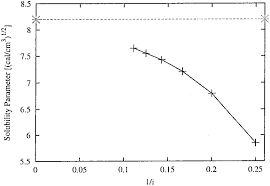The image above represents the standing gas solubility parameter.

To compute for the standing gas solubility parameter, two essential parameters are needed and these parameters are API Gravity (°API) and Temperature (°Rankine) (T).

The formula for calculating the standing gas solubility parameter:

x = 0.0125°API – 0.00091(T – 460)

Where:

x = Standing Gas Solubility Parameter
°API = API Gravity
T = Temperature (°Rankine)

Let’s solve an example;
Given that the API Gravity is 22 and the temperature is 120.
Find the standing gas solubility parameter?

This implies that;

°API = API Gravity = 22
T = Temperature (°Rankine) = 120

x = 0.0125°API – 0.00091(T – 460)
x = 0.0125(22) – 0.00091(120 – 460)
x = 0.275 – 0.00091(-340)
x = 0.275 – -0.3094
x = 0.5844

Therefore, the standing gas solubility parameter is 0.5844.

## How to Calculate and Solve for Standing Gas Solubility of a Fluid | The Calculator Encyclopedia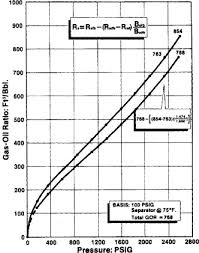The image above represents standing gas solubility.

To compute for the standing gas solubility, three essential parameters are needed and these are Solution Gas Specific Density (γg), System Pressure (P) and Standing Gas Solubility Parameter (x).

The formula for calculating the standing gas solubility:

Standing Rs = γg[(P / 18.2 + 1.4) 10x ]1.2048

Where:

Standing Rs = Standing Gas Solubility
γg = Solution Gas Specific Density
P = System Pressure
x = Standing Gas Solubility Parameter

Let’s solve an example;
Find the standing gas solubility when the solution gas specific density is 12, the system pressure is 40 and the standing gas solubility parameter is 20.

This implies that;

γg = Solution Gas Specific Density = 12
P = System Pressure = 40
x = Standing Gas Solubility Parameter = 20

Standing Rs = γg[(P / 18.2 + 1.4) 10x ]1.2048
Standing Rs = 12[(40 / 18.2 + 1.4) 1020 ]1.2048
Standing Rs = 12[(3.597802197802198) 1020]1.2048
Standing Rs = 12[(3.597802197802198) (100000000000000000000)]1.2048
Standing Rs = 121.2048
Standing Rs = 12[5.833292021408776e+24]
Standing Rs = 6.999950425690531e+25

Therefore, the standing gas solubility is 6.999950425690531e+25.

## How to Calculate and Solve for Total Formation Volume Factor | The Calculator Encyclopedia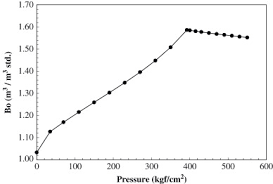The image above represents the total formation volume factor (FVF).

To compute for the total FVF, four essential parameters are needed and these parameters are oil FVF (Bo), gas FVF (Bg), initial gas solubility (Rsi) and current gas solubility (Rs).

The formula for calculating the total FVF:

BT = Bo + (Rsi – Rs)Bg

Where;

BT = Total FVF
Bo = Oil FVF
Bg = Gas FVF
Rsi = Initial Gas Solubility
Rs = Current Gas Solubility

Let’s solve an example;
Find the total FVF when the oil FVF is 14, gas FVF is 15, initial gas solubility is 26 and current gas solubility is 17.

This implies that;

Bo = Oil FVF = 14
Bg = Gas FVF = 15
Rsi = Initial Gas Solubility = 26
Rs = Current Gas Solubility = 17

BT = Bo + (Rsi – Rs)Bg
BT = 14 + (26 – 17)15
BT = 14 + (9)15
BT = 14 + 135
BT = 149

Therefore, the total FVF is 149.

Calculating the Oil FVF when the Total FVF, Gas FVF, Initial Gas Solubility and Current Gas Solubility is Given.

Bo = BT – (Rsi – Rs)Bg

Where;

Bo = Oil FVF
BT = Total FVF
Bg = Gas FVF
Rsi = Initial Gas Solubility
Rs = Current Gas Solubility

Let’s solve an example;
Find the oil FVF when the total FVF is 44, gas FVF is 17, initial gas solubility is 21 and current gas solubility is 19.

This implies that;

BT = Total FVF = 44
Bg = Gas FVF = 17
Rsi = Initial Gas Solubility = 21
Rs = Current Gas Solubility = 19

Bo = BT – (Rsi – Rs)Bg
Bo = 44 – (21 – 19)17
Bo = 44 – (2)17
Bo = 44 – 34
Bo = 10

Therefore, the oil FVF is 10.

Calculating the Gas FVF when the Total FVF, Oil FVF, Initial Gas Solubility and Current Gas Solubility is Given.

Bg = BT – Bo / Rsi – Rs

Where;

Bg = Gas FVF
Bo = Oil FVF
BT = Total FVF
Rsi = Initial Gas Solubility
Rs = Current Gas Solubility

Let’s solve an example;
Find the gas FVF when the total FVF is 48, oil FVF is 16, initial gas solubility is 20 and current gas solubility is 12.

This implies that;

Bo = Oil FVF = 16
BT = Total FVF = 48
Rsi = Initial Gas Solubility = 20
Rs = Current Gas Solubility = 12

Bg = BT – Bo / Rsi – Rs
Bg = 48 – 16 / 20 – 12
Bg = 32 / 8
Bg = 4

Therefore, the gas FVF is 4.